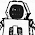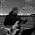## Monday, August 31, 2009

### Quick Dice Average

Quick way to find the average (expected value) of dice xDy: Take half of x, add one to y, then multiply the two. This is equivalent to what some refer to as "Gauss's formula" for summing a series. For example:

8d6 -> 4*7 = 28
12d4 -> 6*5 = 30

1.I ran across this site recently which has been somewhat useful:

http://catlikecoding.com/anydice/

2.Thanks Delta and K. Bailey. Useful stuff.

3.Probably known to all, but it also works for odd x and/or y